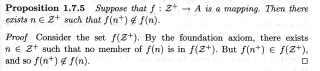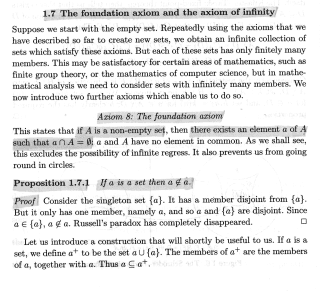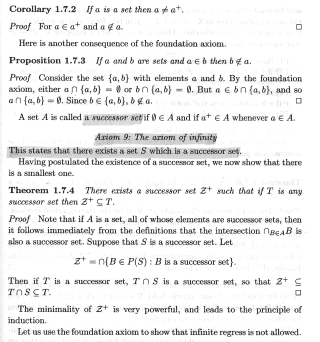# ZFC ... Axioms of Foundation ... and Infinity ...

Gold Member
I am reading D. J. H. Garling's book: "A Course in Mathematical Analysis: Volume 1: Foundations and Elementary Real Analysis" ... ...

I am at present focused on Part 1: Prologue: The Foundations of Analysis ... Chapter 1: The Axioms of Set Theory ...

I need help with an aspect of the proof of Proposition 1.7.5 ...

Proposition 1.7.5 reads as follows:In the above proof we read the following:

"By the foundation axiom, there exists ##n \in \mathbb{Z}^+## such that no member of ##f(n)## is in ##f( \mathbb{Z}^+ )##. ... ... "

Can someone please explain how/why the foundation axiom implies that there exists ##n \in \mathbb{Z}^+## such that no member of ##f(n)## is in ##f( \mathbb{Z}^+ )##. ... ... ?

Peter

NOTE:

To enable readers to follow the above post I am providing Garling's text on the foundation axiom and the axiom of infinity ... ...## Answers and Replies

andrewkirk
Science Advisor
Homework Helper
Gold Member
The claim can be re-stated as:

There exists an element ##n## of ##\mathcal Z^+## such that ##f(n)\cap f(\mathcal Z^+)=\emptyset\quad\quad\quad\quad\quad## (1)

Line up statement (1) next to the statement of the foundation axiom and see if you can find some substitutions to make to the foundation axiom to get (1). The substitution is not one-for-one exact, but the variation from exact substitution should not be too hard to negotiate.

•Math Amateur
Gold Member
Hi Andrew ... sorry to be slow in replying ... had to travel interstate ...

Sorry to be slow Andrew ... but can you explain how ...

the statement that there exists an ##n \in \mathbb{Z}^+## such that ##f(n+) \notin f(n)## ...

is equivalent to ...

the statement that there exists an element ##n \in \mathbb{Z}^+## such that ##f(n) \cap f( \mathbb{Z}^+ ) = \emptyset## ...

Peter

andrewkirk
Science Advisor
Homework Helper
Gold Member
The statement in the text we are trying to match is:
there exists ##n \in \mathcal{Z}^+## such that no member of ##f(n)## is in ##f( \mathcal{Z}^+ )##. ...
That is equivalent to
there exists ##n \in \mathcal{Z}^+## such that ##f(n)\cap f( \mathcal{Z}^+ )=\emptyset##. ...

The foundation axiom is
if ##A## is non-empty then there exists ##a \in A## such that ##a\cap A=\emptyset##. ...

Now substitute ## f( \mathcal{Z}^+ )## for ##A## and ##f(n)## for ##a## in that last one, to get
if ##f( \mathcal{Z}^+ )## is non-empty then there exists ##f(n) \in f( \mathcal{Z}^+ )## such that ##f(n)\cap f( \mathcal{Z}^+ )=\emptyset##. ...
and note that ##\mathcal{Z}^+## is non-empty by definition, hence so is ##f(\mathcal Z^+)##, and that if
• there exists ##f(n)\in f( \mathcal{Z}^+ )## such that ##f(n)\cap f( \mathcal{Z}^+ )=\emptyset##; then
• there exists ##n\in \mathcal Z^+## such that ##f(n)\cap f( \mathcal{Z}^+ )=\emptyset##
since "##\exists n\in\mathcal Z^+##" is logically equivalent to "##\exists f(n)\in f(\mathcal Z^+)##", given that the domain of ##f## contains ##\mathcal Z^+##.

Note also that the Z is ##\mathcal Z##, which is not the integers ##\mathbb Z## (although he may end up identifying it with the positive integers later on). The TeX code around the Z is \mathcal rather than \mathbb.

•Math Amateur
Gold Member
Thanks so much for the help, Andrew ... clear now ...

Peter